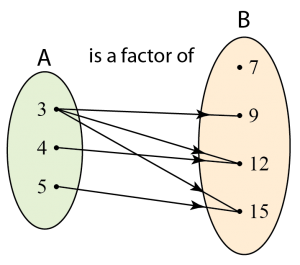\

# 1.1.1 Relation – Domain and Codomain

### Domain and Codomain

1. In the relation between one set and another, the first set is known as the domain and the second set is known as the codomain.
2. Elements in the domain is called objects, whereas elements in the codomain mapped to the objects is called the image.
3. Elements in the codomain not mapped to the objects are not the image.
4. All images in codomain can be written as a set known as range.
Example:Domain = {3, 4, 5}
Codomain = {7, 9, 12, 15}
Range = {9, 12, 15} [Note: 7 is not an image because it is not mapped to any object.]

3 is the object of 9, 12 and 15.
4 is the object of 12.
5 is the object of 15.

9, 12 and 15 are the images of 3.
12 is the image of 4.
15 is the image of 5.